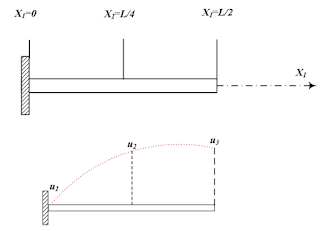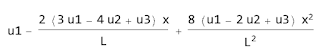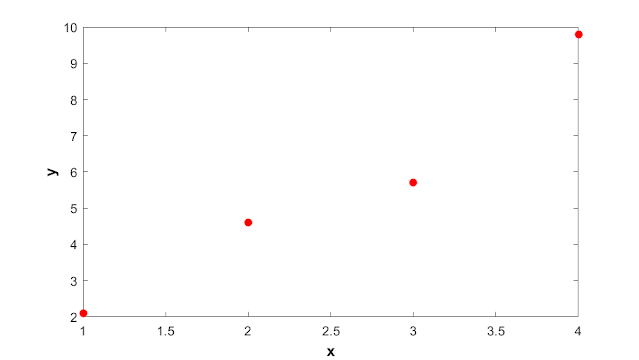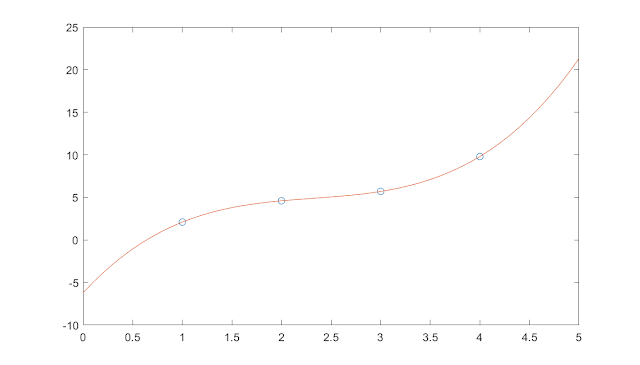## Welcome to the World of Modelling and Simulation

### What is Modelling?

This blog is all about system dynamics modelling and simulation applied in the engineering field, especially mechanical, electrical, and ...### Stress and Strain Analysis of Linear Finite Elements

In this tutorial, we will use principle of virtual work method to determine the stress and displacement of the nodes of linear one dimensional finite elements. Following figure 1 represents a cantilever beam which is stressed axially. Let's assume the parameters L, A, E, c have the same value of 1 unit for simplicity. Our goal is to compare the finite element results with the exact solutions of a typical cantilever problem.
For this problem, we need to consider two quadratic one dimensional element. So, the displacement is interpolated among the three nodes and has the following form:Fig.1: Showing one dimensional axially load bar given in the assignment problem.Fig.2: Showing one dimensional piecewise linear interpolation.

## Mathematica Program for Element 1

Clear[x, a0, a1, a2, u1, u2, u3, L]

(*Definition of quadratic form of displacement*)
u = a0 + a1*x + a2*x^2;

eq1 = (u /. x -> 0) - u1;
eq2 = (u /. x -> L/4) - u2;
eq3 = (u /. x -> L/2) - u3;

sol = Solve[{eq1 == 0, eq2 == 0, eq3 == 0}, {a0, a1, a2}];
u = u /. sol[]

N1 = Coefficient[u, u1];
N2 = Coefficient[u, u2];
N3 = Coefficient[u, u3];

(*Shape Functions*)
Nn = {N1, N2, N3}

p = c*x;

(*Nodal Force Vectors*)
fe = Integrate[Nn*p, {x, 0, L/2}];
c = 1;
L = 1;
fe // MatrixForm

### Program Outputs

Displacement Vector for Element 1:Shape Functions for Element 1:

Nodal Force Vector for Element 1:

## Mathematica Program for Element 2

Clear[x, a0, a1, a2, u1, u2, u3, L]

(*Definition of quadratic form of displacement*)
u = a0 + a1*x + a2*x^2;

eq1 = (u /. x -> 0) - u1;
eq2 = (u /. x -> L/4) - u2;
eq3 = (u /. x -> L/2) - u3;

sol = Solve[{eq1 == 0, eq2 == 0, eq3 == 0}, {a0, a1, a2}];
u = u /. sol[]

N1 = Coefficient[u, u1];
N2 = Coefficient[u, u2];
N3 = Coefficient[u, u3];

(*Shape Functions*)
Nn = {N1, N2, N3}

p = c*(x + L/2);

(*Nodal Force Vectors*)
fe = Integrate[Nn*p, {x, 0, L/2}];
c = 1;
L = 1;
fe // MatrixForm

### Program Outputs

Displacement Vector for Element 2

Shape Functions for Element 2

## Local Stiffness Matrix for Element 1

Clear[x, a0, a1, a2, u1, u2, u3, L, EA]

(*Definition of quadratic form of displacement*)
u = a0 + a1*x + a2*x^2;

eq1 = (u /. x -> 0) - u1;
eq2 = (u /. x -> L/4) - u2;
eq3 = (u /. x -> L/2) - u3;

sol = Solve[{eq1 == 0, eq2 == 0, eq3 == 0}, {a0, a1, a2}];
u = u /. sol[];

N1 = Coefficient[u, u1];
N2 = Coefficient[u, u2];
N3 = Coefficient[u, u3];

B = {{D[N1, x], D[N2, x], D[N3, x]}};

(*Local Stiffness Matrix*)
K = Integrate[EA*Transpose[B].B, {x, 0, L/2}];

L = 1;
EA = 1;
K // MatrixForm

## Comparison between the Exact and Solution using Four Elements

We know that the exact solution for the axially loaded bar shown in Fig. 1 may be written as,

Where, for the consecutive four elements, we may write the displacement for each element as,

From the assignment problem, the parameter values are,

From the virtual work method, we may write,

The internal virtual work (IVW) may be expressed as,

And, the external virtual work may be presented as,

## MATHEMATICA PROGRAM

The following program is written to determine the nodal displacement using the finite element method considering the principle of virtual work.

Clear[x, a0, a1, a2, u1, u2, u3, L]

(*Definition of quadratic form of displacement*)
u = a0 + a1*x + a2*x^2;

eq1 = (u /. x -> 0) - u1;
eq2 = (u /. x -> L/4) - u2;
eq3 = (u /. x -> L/2) - u3;

sol = Solve[{eq1 == 0, eq2 == 0, eq3 == 0}, {a0, a1, a2}];
u = u /. sol[];

(*Shape Functions*)
N1 = Coefficient[u, u1];
N2 = Coefficient[u, u2];
N3 = Coefficient[u, u3];
Nn = {N1, N2, N3};

B = {{D[N1, x], D[N2, x], D[N3, x]}};

c = 1;
L = 1;
EA = 1;

(*Axial Force on Element 1*)
p1 = c*x;
fe1 = Integrate[Nn*p1, {x, 0, L/2}];

(*Axial Force on Element 2*)
p2 = c*(x + L/2);
fe2 = Integrate[Nn*p2, {x, 0, L/2}];

K1 = Integrate[EA*Transpose[B].B, {x, 0, L/2}];

K2 = Integrate[EA*Transpose[B].B, {x, 0, L/2}];

Ndof = 5;
Ne = 2;
ID1 = {1, 2, 3};
ID2 = {3, 4, 5};

K = ConstantArray[0, {Ndof, Ndof}];
K[[ID1, ID1]] = K1 + K[[ID1, ID1]];
K[[ID2, ID2]] = K2 + K[[ID2, ID2]];

fe = ConstantArray[0, {Ndof, 1}];
fe[[ID1]] = fe1 + fe[[ID1]];
fe[[ID2]] = fe2 + fe[[ID2]];

IDE = {1};
IDN = {2, 3, 4, 5};

KR = Delete[K, IDE];
KR = Delete[Transpose[KR], IDE];
KR = Transpose[KR];

feR = Delete[fe, IDE];

(*Calculation of Nodal Displacements*)
DisR = LinearSolve[KR, feR];

Dis = ConstantArray[0, {Ndof, 1}];
Dis[[IDN]] = DisR + Dis[[IDN]];
Dis // MatrixForm

### Program Outputs

So, we see that the exact and finite element solutions are the same.

From the following two plots, we notice that although the nodal displacement is continuous from the FEA result, it is not true for the stress. The stress distribution is indeed discontinuous.

## MATHEMATICA PROGRAM FOR COMPARISON

(*Exact Solution*)
uexact = c*L^2/2/EA*x - c/6/EA*x^3;

(*Finite Element Approximation*)
N1 = Piecewise[{{4 x/L, 0 <= x <= L/4}, {4/L (L/2 - x),
L/4 <= x <= L/2}}, 0];
N2 = Piecewise[{{4/L (x - L/4), L/4 <= x <= L/2}, {4/L (3 L/4 - x),
L/2 <= x <= 3 L/4}}, 0];
N3 = Piecewise[{{4/L (x - L/2), L/2 <= x <= 3 L/4}, {4/L (L - x),
3 L/4 <= x <= L}}, 0];
N4 = Piecewise[{{4/L (x - 3 L/4), 3 L/4 <= x <= L}}, 0];

u1 = c*L^3/EA*(47/384);
u2 = c*L^3/EA*(11/48);
u3 = c*L^3/EA*(39/128);
u4 = c*L^3/EA*(1/3);

(*Calculating Nodal Displacement*)
u = u1*N1 + u2*N2 + u3*N3 + u4*N4;
uexactp = uexact /. {c -> 1, EA -> 1, L -> 1}

sigmaexact = D[uexactp, x]
up = u /. {c -> 1, EA -> 1, L -> 1}
sigmap = D[up, x]

(*Plotting to Show the Comparison between Exact and FEA Solution*)
Plot[{uexactp, up}, {x, 0, 1},
AxesLabel -> {"x", "Nodal Displacement"},
PlotLegends -> {"uexact", "u_Finite Element"}]
Plot[{sigmaexact, sigmap}, {x, 0, 1}, AxesLabel -> {"x", "sigma_11"},
PlotLegends -> {"sigma_exact", "sigma_Finite Element"}]

#FiniteElement #StressStrain #Mathematica #Abaqus #FEA #Blog #Blogger

### Some Frequently used Interpolation Tools by MATLAB

Interpolation is a very essential tool in any areas of research these days. Whether you are analyzing your data or deriving equations to represent a system, interpolation plays an immense role in these works. Luckly, MATLAB has some built-in commands for basic data fitting. Not only that, you may also create your own function and use it stand alone or in combination of calling some MATLAB commands. In this article, I am going to discuss three basic techniques for basic data fitting. They are,

• Polynomial Interpolation
• Piecewise Linear Interpolation
• Cubic Spline Interpolation

Polynomial Interpolation: Polynomial interpolation is one of the most basic interpolation tools. The first step would be to learn this technique manually as it would provide us insights into the fundamentals prior to using MATLAB built-in features. The idea is that a polynomial of n degree would pass through all the (n+1) points if we apply the technique of polynomial interpolation. Let us begin with an example to strengthen the concept.
Let’s say, we have data points: (1, 2.1), (2, 4.6), (3, 5.7) and (4, 9.8). The goal is to fit a polynomial that would pass through all these points. We may consider these points as (x₁, y₁), (x2, y2), (x3, y3), and (x4, y4) where x refers to the horizontal axis and y refers to the vertical axis.Data points from the exampleNow, let's define a general polynomial equation. The following is the n-th order polynomial:If we extend the general polynomial, it looks like the following as,And, in matrix form, we may arrange these equations nicely as follows, For our example problem, we have 4 data points, which means that we will have four equations. The right side vector b is for the vertical (y-axis) data points. The left side nxn matrix is for the horizontal (x-axis) data points. But, there is a question. The horizontal data points are not in a squared matrix, specifically, it is now in 1x4 form; so, how could we represent the data as in a 4x4 matrix for our example? The following figure shows the current configuration:We can write a simple MATLAB program to make that 4x4 matrix. The following codes will generate a square matrix from a single row matrix.% Generation of a Polynomial Matrixx=[1 2 3 4]; % Sample x data pointsn = length(x); A = zeros(n,n); for i=1:n% Creating polynomial matrixfor j=1:n% nth degree polynomial passing through (n-1) pointsA(i,j) = x(i).^(j-1);endenddisp(A)After implementing the above program, we will have the following refined equations, which we can solve by Gaussian elimination to find the value of the unknown coefficients, a.Now, using the Gaussian elimination, the unknown parameters are,So, the polynomial equation that will pass through all the four points is,If we plot, the above equation fits and passes through all the points. So far, the mathematics behind polynomial interpolation has been discussed. Good news! MATLAB has a built-in polynomial interpolation feature. We can use the feature easily. You can access the built-in function from the command prompt shown as below,>> x = [1; 2; 3; 4];>> y =[2.1; 4.6; 5.7; 9.8];>> polyfit(x,y,3)ans =-6.212.667-5.100.7333'polyfit' is the built-in command in MATLAB that uses the same technique shown earlier. Piecewise Linear Interpolation: When we have more data points to fit, polynomial interpolation does generate too much oscillations in curve fitting. In this case, we may use the MATLAB built-in command 'interp1' for one dimensional piecewise linear interpolation. For our four data points example, we may write few lines in MATLAB command prompt to access this feature.>> x1 = [1;2;3;4];>> y1 =[2.1; 4.6; 5.7; 9.8];>> xx = 0:0.1:5;yy = interp1(x1,y1,xx);plot(x1,y1,'o',xx,yy)Cubic Spline Interpolation: An improvement to one dimensional piecewise linear interpolation is the cubic spline interpolation. We may use the MATLAB built-in command 'spline' for it. For our four data points example, we may write few lines in MATLAB command prompt to access this feature.>> x1 = [1;2;3;4];>> y1 =[2.1; 4.6; 5.7; 9.8];>> xx = 0:0.1:5;yy = spline(x1,y1,xx);plot(x1,y1,'o',xx,yy)#Interpolation #PiecewiseLinear #CubicSpline #Matlab #Polynomial #Blog #Blogger

### Vectorization in MATLAB: Use of Colon (:)

We are familiar with 'for loop' application in MATLAB. We may use many 'loops' to handle complex calculations. But, sometimes too many 'loops' cost computational time, which eventually affects the overall efficiency of the codings. In MATLAB, instead of looping, we may use the method of vectorization to skip some 'loops'. We may use the operator colon (:) in MATLAB to form vectors that alternately eliminates the 'loops'. Let's explain the method with a very simple example. For example, we would like to divide a square matrix (for our example, 5x5) with a scalar number. Below, there are three scenarios. At first, we can use 2 'for loops' to do the operation. Secondly, we can skip one 'for loop' by using a colon (:) for the columns of the matrix a. Finally, we can avoid all 'for loops', and use colons (:) for both rows and columns of the example matrix a.

2 'for loop':

i=1;
j=1;
n=5;
a=[1 2 3 4 5; 6 7 8 9 10; 1 2 3 4 5; 6 7 8 9 10; 1 2 3 4 5;];
for i=1:n
for j=1:n
a(i,j) = a(i,j)./n;
end
end
disp(a)

1 'for loop':

i=1;
j=1;
n=5;
a=[1 2 3 4 5; 6 7 8 9 10; 1 2 3 4 5; 6 7 8 9 10; 1 2 3 4 5;];
for i=1:n

a(i,j:n) = a(i,j:n)./n;

end
disp(a)

0 'for loop':

i=1;
j=1;
n=5;
a=[1 2 3 4 5; 6 7 8 9 10; 1 2 3 4 5; 6 7 8 9 10; 1 2 3 4 5;];

a(i:n,j:n) = a(i:n,j:n)./n;

disp(a)

In all the above cases, the results are the same. You may run the codes and check for yourself. The operation is pretty straightforward here. When colon (:) is first used between j and n,  we eliminate a 'for loop'. This colon (:) is considering all the elements in columns of the matrix a, which is equivalent to the 'loop' operation. The second colon (:), between i and n (i:n), takes all the row elements of the matrix a, and the operation is also same compared to using a 'for loop'. Below is the result for all three demonstrated cases.

0.2000    0.4000    0.6000    0.8000    1.0000
1.2000    1.4000    1.6000    1.8000    2.0000
0.2000    0.4000    0.6000    0.8000    1.0000
1.2000    1.4000    1.6000    1.8000    2.0000
0.2000    0.4000    0.6000    0.8000    1.0000

This is a very simple example, and for this, you won't even realize the actual computational efficiency. But, imagine! you have very large matrices where numerous complex operations involved. In that case, you would be able to see the differences.

#Vectorization #Matrix #ForLoop #UseofColon #Matlab #Blog #Blogger

### Comparison between 4 and 8 Node Integration Elements in Finite Element Analysis

Let us assume a cantilever beam with one end fixed and the other end has 1 unit load acting vertically downward. The length and height of the beam are 5 and 1 unit respectively. The Young's modulus is 10,000 unit. At first, we will solve this problem analytically using the famous Euler-Bernoulli beam equation to find the deflection at the end of the beam. Next, we will use Abaqus with 4 node quadrilateral  reduced integration element. Then, we will solve the same problem using 8 node quadrilateral reduced integration element. Our goal is to see which element performs better in terms of convergence and accuracy for the finite element analysis (FEA) compared to the analytical solution.

The following Mathematica program solves the Euler-Bernoulli equation for the deflection at the tip.

(*Exact Solution of Euler-Bernoulli Cantilever Beam*)
Clear[y, P, x, X2, EI, L];
y[x];

(*Given Property Values*)
L = 5;
h = 1;
P = 1;
Ie = 1/12;
Ee = 10000;
EI = Ee*Ie;
theta = D[y[x], x];
V = EI*D[y[x], {x, 3}];
M = EI*D[y[x], {x, 2}];
y1 = y[x] /. x -> 0; y2 = y[x] /. x -> L; theta1 =
theta /. x -> 0; theta2 = theta /. x -> L; M1 = M /. x -> 0; M2 =
M /. x -> L;
V1 = V /. x -> 0; V2 = V /. x -> L;

(*Solving the Equation*)
s = DSolve[{EI*y''''[x] == 0, y1 == 0, theta1 == 0, V2 == P, M2 == 0},
y, x];
Print["Analytical Solution of the Exact Displacement"]
y = Simplify[y[x] /. s[]]
Plot[y, {x, 0, 5}, PlotStyle -> {Blue, Thick}]
Print["Displacement Variation at Point A in both X and Y Direction"]
DA = y /. x -> 5;
theta = D[y, x] /. x -> L;
a1 = N[L - (-h/2)*Sin[theta]];
a2 = N[DA + (-h/2)*Cos[theta]];
A1 = a1 - 5
A2 = a2 + h/2
Print["The Stress at Point B, S11"]
S11 = -Ee*X2*D[y, {x, 2}];
StressatB = S11 /. {x -> 0, X2 -> 0.5}

Now, we begin to simulate the same problem with two types of elements: 4 and 8 node quadrilateral reduced integration. As in any FEA simulation, we are required to define the meshing parameters and elements prior to the analysis, and these two elements are frequently used in Abaqus.

The above results highlight that there is a trend of increasing convergence of the results starting from the fourth elements. We see that the stresses have minute changes in its absolute values, which are also visible for the displacements. With the increase of the element numbers, the faster the different plots to converge. It is evident that the elements are not converging to the same solutions always. Moreover, for this problem, the rate of convergence and accuracy is better with the 8 node quadrilateral reduced integration element; however, with the increase in number of elements, both 4 and 8 node quadrilateral reduced integration elements have similar performance.
If you would like to gather more knowledge on FEA, I would encourage you to visit this site as this tutorial is based on the discussions and examples of that.

### Implementation of Newton Raphson and Modified Newton Raphson Method by Mathematica

This article discusses the Mathematica approaches to code Newton Raphson (NR) and Modified Newton Raphson (MNR) and the differences if any in computation. Let's assume that stress and strain of a rigid bar is defined by the following relationship:

𝜎 = 100000 (√𝜀 + (1/80) sin (𝜋𝜀/0.002))

Now, taking the first derivative of the above relationship, we may write,

𝜎' = 100000 ((1/2√𝜀) + 19.653 cos (1570.80𝜀))

The NR method implements an iterative approach by considering a tangent to a curve, for which the function under consideration becomes zero. If f(xⱼ) is any function and f′(xⱼ) is the derivative of that function at point xⱼ, then according to NR approach, we may write as,

xⱼ₊₁ = xⱼ - f(xⱼ) / f′(xⱼ)

The MNR works in a similar way, but is helpful for converging a solution efficiently for roots with multiplicity and may be represented as,

xⱼ₊₁ = xⱼ - ⟮ f(xⱼ)  f′(xⱼ)⟯ /⟮ f′(xⱼ)² -  f(xⱼ) f′′(xⱼ)⟯

The following Mathematica program is developed to plot the function and its derivatives.

(*Function Defined in the Problem*)
sigma = 100000*(Sqrt[epsilon] + 1/80*Sin[Pi*epsilon/0.002]);
y1 = 4000;
y2 = 6000;
sigmap = D[sigma, epsilon];
sigmap2 = D[sigma, {epsilon, 2}];

(*Plotting the Function, its Derivatives, Upper and Lower Bounds*)
A1 = Plot[{sigma, y1, y2}, {epsilon, 0, 0.005}, ImageSize -> Large,
AxesLabel -> {"Epsilon", "Sigma"}, PlotStyle -> {Red, Green, Black, Thick}]
A2 = Plot[sigmap, {epsilon, 0, 0.005}, ImageSize -> Large,
AxesLabel -> {"Epsilon", "Sigma'"}, PlotStyle -> {Red, Thick}]
A3 = Plot[sigmap2, {epsilon, 0, 0.005}, ImageSize -> Large,
AxesLabel -> {"Epsilon", "Sigma''"}, PlotStyle -> {Red, Thick}]

(*Initial Guesses*)
sigma /. epsilon -> 7.928*10^-4
sigma /. epsilon -> 7.924*10^-4
sigma /. epsilon -> 3.882*10^-3
sigma /. epsilon -> 3.879*10^-3

Mathematica Program for the NR Method:

(*Mathematica Codes for Newton-Raphson Approach*)
Sigma = 100000*(Sqrt[epsilon] + 1/80*Sin[Pi*epsilon/0.002]) - 4000;
epsilontable = {0.0002};
Error = {1};
SetTolerance = 0.0005;
MaximumIteration = 100;
i = 1;

(*Newton-Raphson Algorithm Implementation*)
While[And[i <= MaximumIteration, Abs[Error[[i]]] > SetTolerance],
epsilonnew =
epsilontable[[i]] - (Sigma /.
epsilon -> epsilontable[[i]]);
epsilontable = Append[epsilontable, epsilonnew];
Errornew = (epsilonnew - epsilontable[[i]])/epsilonnew;
Error = Append[Error, Errornew];
i++];

L = Length[epsilontable];
SolutionTable =
Table[{i - 1, epsilontable[[i]], Error[[i]]}, {i, 1, L}];
SolutionTable1 = {"Iteration Number", "Epsilon", "Error"};
L = Prepend[SolutionTable, SolutionTable1];
Print["Showing Results for the Newton-Raphson Approach when Sigma is \
4,000 and Initial Guess is 0.0002"]
ScientificForm[L // MatrixForm, 4]

Mathematica Program for the MNR Method:

(*Mathematica Codes for the Modified Newton-Raphson Approach*)
Sigma = 100000*(Sqrt[epsilon] + 1/80*Sin[Pi*epsilon/0.002]) - 4000;
epsilontable = {0.0002};
SigmaDerivative = D[Sigma, epsilon] /. epsilon -> epsilontable[];
Error = {1};
SetTolerance = 0.0005;
MaximumIteration = 100;
i = 1;

(*Implementation of the Modified Newton-Raphson Algorithm*)
While[And[i <= MaximumIteration, Abs[Error[[i]]] > SetTolerance],
epsilonnew =
epsilontable[[i]] - (Sigma /. epsilon -> epsilontable[[i]])/
Errornew = (epsilonnew - epsilontable[[i]])/epsilonnew;
Error = Append[Error, Errornew];
i++];

T = Length[epsilontable];
SolutionTable =
Table[{i - 1, epsilontable[[i]], Error[[i]]}, {i, 1, T}];
SolutionTable1 = {"Iteration Number", "Epsilon", "Error"};
T = Prepend[SolutionTable, SolutionTable1];
Print["Showing Results for the Modified Newton-Raphson Approach when \
Sigma is 4,000 and Initial Guess is 0.0002"]
ScientificForm[T // MatrixForm, 4]

Results:

The following table shows the comparison of results from NR and MNR methods. We see that at iteration number 17, the Modified Newton-Raphson approach converges, whereas the Newton-Raphson approach is still iterating. However, it is noticeable that the step is faster than the Modified approach. Nevertheless, Newton-Raphson approach fails to converge early while the Modified approach, although iterates slowly, but converges eventually.

#NewtonRaphson #Mathematica #MatrixForm #Blog #Blogger

### Discussion on MATLAB Function and Function Handle

In MATLAB, the functions and function handles (@) are used frequently, especially when writing codes in m-files. We can create many functions, but when we call a particular function, and if that function has another function inside the input parameters - the function handle (@) is the way to address and call the second function. For example, we have created a function to find the roots of a polynomial using the Newton-Raphson method and named it as 'newtonraphson'. The function input parameters are: polynomial, initial guess, error tolerance and maximum number of iterations. And the output is the roots of the polynomial.

Now, to define the polynomial, we can create another function and name it as 'polyfunction'. This function only has the polynomial, and we are interested to find its roots. Let's see how we can call the function 'newtonraphson' in MATLAB command prompt.

>> newtonraphson(@ polyfunction, 1.0, 1e-5, 100)

Here,

newtonraphson = a function for finding roots of a polynomical
@ = function handle to call another function
1.0 = Initial guess
1e-5 = Error tolerance
100 = Maximum number of iterations

If you do not put the function handle (@) in front of the 'polyfunction', there will be an error you will see instantly in the command prompt. As you can see, a function handle is used to pass information to another function. There are other uses of the function handle in MATLAB, but the above is most frequently and widely used expression.

The aforementioned example shows the application of the function handle (@) to a known or defined function by the user (e.g. 'polyfunction'). Now, if there is an anonymous equation that we need to define in our coding, then we can also use the function handle to do the job. For example, in our program, we need to define an equation: 3x - 4y + 2z. The x, y, and z are the unknowns. To define it with the function handle, we can write as,

>> L = @ (x, y, z) (3*x - 4*y + 2*z)

Here,

L = is any parameter referring the equation
@ = function handle defining x, y, z with respect to the equation for evaluation

#MatlabFunction #FunctionHandle #PolyFunction #NewtonRaphson #Matlab #Blog #Blogger